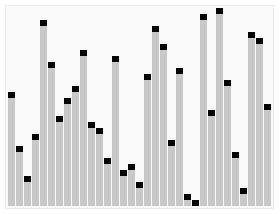# Tractable / Intractable Problem

## Type

### Tractable

the problems that can be solved by a computer using no more time than some slowly growing function of the size of the input are called tractable

### Intractable

• Problems that (appear to) require exponential time.
• NP-completeness and beyond.

intractability: Solvable Problems but with an exponential time in the input size.

The solution must then be approximated.

While the undecidable problems have been proved not to have any solution, for the intractable problems we have very strong evidence that they require exponential time, but no proof.

Discover MoreAlgorithm - NP Complete (Nondeterministic Polynomial Time Complete)

This is a class of problems that are so difficult that even the best solutions cannot consistently determine their solutions in an efficient way. Specifically, NP Complete problems can only possibly...Algorithm - Problem

A problem in algorithm is what need to be solved. Problems that cannot be solved by computation are called undecidable Problems that can be solved by computer are called decidable Tractable:...Automaton (State Machine)

Automaton (State Machine) Automata theory is the study of abstract computing devices or machines. The word automata (the plural of automaton) comes from the Greek word αὐτόματα, which means...Polynomial time algorithms

polynomial time algorithm. A problem that: can be solved in polynomial time is Tractable can NOT be solved in polynomial time is intractable A function f is a one-way if and only if it can...Turing Machine

are automata that model the power of real computers They allow: the study of decidabilty to distinguish tractable problems